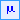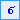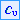Join one of our upcoming courses taking place around the world! Explore now

# Show Values

When you view probabilistic analysis results in Interpret you will notice the following options in the Statistics menu and the toolbar:

•Show Base File Values
•Show Mean Values
•Show Standard Deviation Values
•Show Coefficient of Variation Values

These options allow you to choose the statistical data you would like to display on the contour plots, as described below.

## Show Base File Values

The Show Base File Values option displays the data computed for the base file.

By default, when you open a probabilistic analysis file in RS2, the Base File is the file with the mean input data values.

For example if your model uses two random variables:

• Cohesion (Mean = 5, Std. Dev. = 1)
• Friction Angle (Mean = 30, Std. Dev. = 3)

when you open the file in Interpret, the Base File will be the file with input values Cohesion = 5 and Friction Angle 30.

When you are viewing the Base File contour data, just remember that you are viewing the data from a single file - the Base File.

## Show Mean Values

The Show Mean Values option will display the mean results averaged from ALL of the statistical component files.

For example if your model uses two random variables, the point estimate method will generate FOUR combinations of random input data and therefore FOUR component files for the probabilistic analysis. The Show Mean Values option will display contours of analysis results obtained by averaging the data from all four component files.

## Show Standard Deviation Values

The Show Standard Deviation Values option will display the standard deviation of the results computed from all probabilistic component files.

## Show Coefficient of Variation Values

The Show Coefficient of Variation Values option will display the coefficient of variation of the results computed from all probabilistic component files.